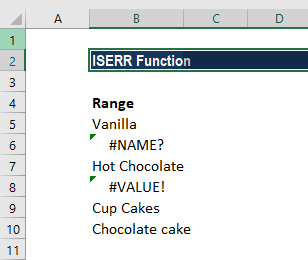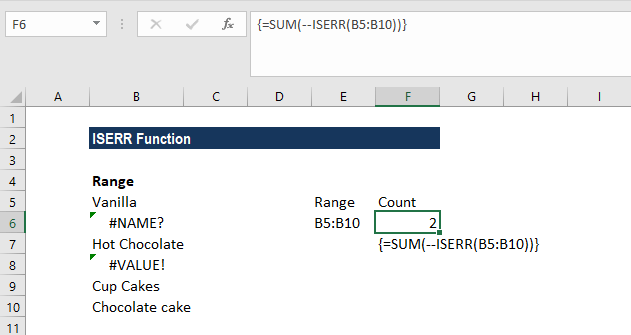# ISERR Function

Returns TRUE if the given value is an error (except N/A) and vice versa

## What is the ISERR Excel Function?

The ISERR Excel Function is categorized under Information functions. The function will return TRUE if the given value is an error (except N/A) and FALSE if it is not. It belongs to the IS group of functions, which includes ISERROR, IFERROR, etc. ISERR is used in combination with the IF function to identify a potential error in a formula and display other formulas or text strings in message form or blanks. It can also be used with the IF function to display a custom message or perform some other calculation if an error is found.

In financial analysis, we need to deal with formulas and data. Often, our spreadsheet will contain a large number of formulas. Sometimes, they won’t work properly to do calculations as required when an error is encountered. The ISERR function, in combination with the IF function, can be used to default a cell’s value when an error is encountered. It allows formulas to work and evaluate data properly without requiring the user’s intervention.

### Formula

=ISERR(value)

The ISERR Excel function uses the following argument:

1. Value (required argument) – This is the expression or value that needs to be tested. It is generally provided as a cell address.

### How to use the ISERR Excel Function?

To understand the uses of the function, let’s consider a few examples:

#### Example 1

Let’s see the results from the ISERR Excel function when we provide the following data:

DataFormulaResultRemarks
210=ISERR(210)FALSEAs there is no error, so FALSE
#REF!=ISERR(#REF!)TRUETRUE, as it is an error
#N/A=ISERR(#NA)FALSEFALSE, as it doesn’t take N/A
25/0=ISERR(25/0)TRUETRUE, as the formula is an error
#VALUE!=ISERR(#VALUE!)TRUETRUE, as it is an error
=ISERR()FALSENo error, so FALSE

#### Example 2

If we wish to count the number of cells that contain errors, we can use the ISERR function wrapped in the SUM function.

Suppose we are given the following data:Using the formula =SUM(–ISERR(B5:B10)), we can get the count of cells with an error, as shown below. Remember to put this formula in an array. For the array, we need to press Control + Shift + Enter instead of just Enter.In the above formula:

1. The SUM function accepted one or more arrays and calculated the sum of products of corresponding numbers. As we provided only one array, it just summed up the items in the array.
2. ISERR now evaluates each of the cells in the given range. The result would be FALSE, TRUE, FALSE, TRUE, FALSE, FALSE.
3. As we used double unary, the results TRUE/FALSE were transformed to 0 and 1. It made the results look like {0.1,0,1,0,0}.
4. SUM summed up the items in the array and returned the total, which is 2.
5. An alternative to the formula above is to use the SUMPRODUCT function. The formula to use will be =SUMPRODUCT(–ISERR(range)).

### Notes about the ISERR Excel Function

1. Use the function to see if a cell contains an error except for #N/A. It includes #VALUE!, #REF!, #DIV/0!, #NUM!, #NAME?, or #NULL! errors.
2. It ignores #N/A, so if we want to also count #N/A, we can use the ISERROR function instead of ISERR.
3. If we wish to provide a custom message, for example, instead of getting a #DIV/0 error, we want the value to be 0, then we can use the formula =IF(ISERR(A4/B4),0,A4/B4) instead of A4/B4.

Thanks for reading CFI’s guide to important Excel functions! By taking the time to learn and master these functions, you’ll significantly speed up your financial analysis. To learn more, check out these additional CFI resources:

• Excel Functions for Finance